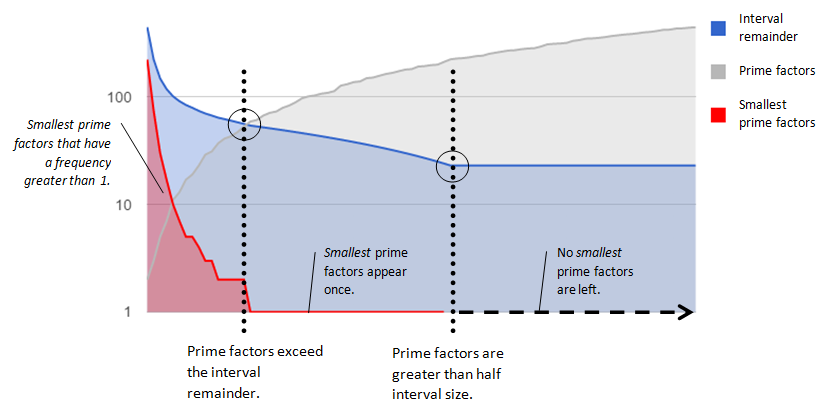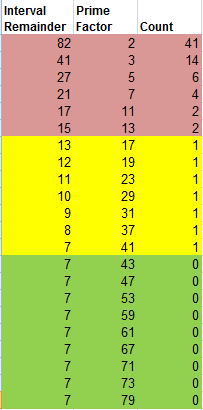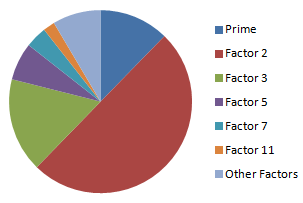Why Is p∈{x to 2x} Nearly Equivalent to p∈{x2 to (x+1)2 }?

The following paper explains why the prime count for any given x to 2x is nearly equivalent to the prime count for x^2 to (x+1)^2. The relationship can be illustrated thus:In this representation of interval 81–100 notice that the greater-than-half interval primes 11, 13, and 17 are prime factors once or twice in this interval (11*3*3, 11*2*2*2, 13*7, and 17*5).

Anatomy of an Interval

You can visualize an interval’s “anatomy” this way:From the foregoing example, you would place 11, 13, and 17 in the graph as “prime factors greater than half the interval size (and less than the whole interval size)”. Because they are greater than half the interval, they must be the largest prime factors of their composites. All the other primes in the interval - the ones less than half - can be the smallest prime factors of their composites. This is the key to the explanation that follows.

The explanation for this rough equivalency rests upon the integer ratios within any interval between two square numbers: (x+1)2 - x2. Observe that, in fact, any even span of numbers you select represents a perfect square interval.

Before proceeding, note that:

• Smallest Prime Factor means an individual composite’s smallest prime factor (such as 7 in  29 * 23 * 7 = 4669)
• The Interval Size does not include the interval endpoints, x2 and (x+1)2. Therefore, every interval size is an even number. (The interval size is twice the square root of the first perfect square.)

Interval-Integer Distribution

For any interval, consider the smallest prime factor (SPF) of every composite. We are interested in the frequency distribution of SPFs within the interval. Each SPF is an element of the interval without considering the composite(s) to which it belongs. We should state the obvious that no SPF can be greater than half the interval size.Example

Perfect Squares: 1681 - 1764  (Interval size: 82)

The following table shows the number of times each prime less than the interval size occurs as the smallest prime factor in the composites of the interval.Less than half the intervalThese primes occur as SPFs in a precise ratio (see below): their frequency is the interval remainder multiplied by their inverse. Less than half the interval, more than the interval remainderI’m not certain if the SPF count can be anything but 0, 1, or 2. (Assigning a count of 1 to each one is an overestimate for the group.) More than half the intervalThese primes cannot occur as SPFs in the interval. (They can appear once or twice as the largest factor.)

Let’s look at the three components of the interval-integer distribution in further detail:

1. Less than half the interval
The frequency (occurrence or count) of the smallest prime factor in each composite less than half the interval is precisely defined.

Divide the total number of integers by each prime and carry forward only the remainder (remaining integers) for the next prime until the primes are greater than the remainder.

This distribution is constant for any arbitrary span of integers – in this case, 10,000 – like this:

 Prime Factor Count Remainder 2 5000 4999 3 1665 3333 5 666 2667 7 380 2286 11 207 2079 All Other Factors 852 Primes 1229Visualized as a pie chart, note that 3 is a third of one-half, 5 is a fifth of two-thirds, and so on.  (The pie is completed by the primes.)

1. Less than half the interval, more than the interval remainder
The intermediate smallest prime factors are less than half the interval but greater than the calculated interval remainder. All these must be represented at least once as SPFs.

Type 1 and 2 SPFs are prime factors of every composite in the interval. That is, factoring any composite will yield at least one such SPF.

1. More than half the interval
These prime factors can occur once or twice in the interval, but never as a composite’s SPF. When they occur in composites, there is always a type 1 or 2 SPF. Their contribution to composites is fully accounted for by the less-than-half-interval prime factors.

Setting the Lower Bound

By considering every interval as containing a frequency distribution of prime factors, we understand that all composites contain - are factorized by - SPFs less than half the interval.

Let’s add together every occurrence of prime factors less than half. In the example, that’s 41+14+6+4+2+2+1+1+1+1+1=76. This number is the maximum number of integers that can be composite. If we subtract that from the interval size of 82, we have a remainder of 6. This number is the minimum number of integers that must be prime. Yes, it is that simple.

Now we need to understand the meaning of the greater-than-half-interval prime factors within this interval frequency distribution.

The “Slot Machine”: Why There Must Always Be Another Prime

The difficulty in proving there must be at least one prime in every interval is in eliminating the possibility that every integer in an interval could be composite. If composites consisted only of distinct prime factors such a possibility would probably not need to be considered. However, nondistinct prime factors suggest that, combinatorially, such a situation could arise.

I can explain why this is, in fact, arithmetically impossible, but I can only do this using a metaphor of “slots”. Think of it like Hilbert’s Hotel, except there are a finite number of slots instead of an infinite number of rooms.

Recalling 1681 - 1764, imagine that every prime in this 82-integer interval - from 2 to 79 - is lined up in a row. There’s a slot for each one, but in order to occupy the slot it must be the smallest prime factor of at least one composite.

Slots can accommodate more than one composite. The smaller the prime, the more composites its slot contains. So following the deterministic frequency distribution, 2 must have 41 composites in its slot, 3 must have 14, 5 must have 6, and so on.

Now it turns out that the primes that are greater than half the interval cannot have slots of their own because they can never be smallest prime factors. They’re already been taken by a smallest prime factor in one of the already occupied slots. Their composites do not need their own slots.

This leaves vacant slots that have no composite occupants. Those are “reserved” for primes! Now look at the graphic.The unfilled slots are integers that must be filled by primes.

This is why the number of primes  between x2 and (x+1)2 must correlate with the number of prime factors > x(x+1) and < (x+1)2.

Are there always empty slots?

Yes, there are always empty slots. That’s because there is always at least one prime that is x < p < 2x. There must be, ipso facto, a subset of prime factors that are greater than half an interval. We see these being “brought forward” by every successive interval. All the previously occurring prime factors, from  2, are still in the fractional equation for every successive interval.

The largest prime factor of any interval will be a greater-than-half-interval prime factor for every subsequent interval until the interval size doubles.

So, for example, 79 is a greater-than-half prime factor from interval 82 to interval 164. To state the obvious, that’s 82 successive perfect square intervals. This interval-span increase for each successive largest prime factor must also extend indefinitely.

Therefore, if the relationship stated in this document is correct, prime count per interval must extend infinitely.